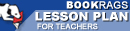Lesson Plans

# The Logic of Scientific Discovery Quiz | Two Week Quiz A

This set of Lesson Plans consists of approximately 102 pages of tests, essay questions, lessons, and other teaching materials.
 View a FREE sampleName: _________________________ Period: ___________________

This quiz consists of 5 multiple choice and 5 short answer questions through Part II: Chapter 6, Degrees of Testability.

## Multiple Choice Questions

1. What must be identical to basic statements?
(a) Theories.
(b) Universal statements.
(c) Singular statements.
(d) Potential falsifiers.

2. What does the principle of causality say about all events?
(a) They must use previous assumptions.
(b) They must be falsifiable.
(c) They can in inductively predicted.
(d) They can be deductively predicted.

3. What confuses the distinction between universal and individual names?
(a) Theoretical statements.
(b) Existential statements.
(c) Symbolic logic.
(d) Negative statements.

4. What denotes what is universal about an occurrence?
(a) Post events.
(b) Sub-events.
(c) Singular statements.
(d) Events.

5. What does Popper do if proof for a statement can not be found?
(a) Suspends judgment.
(b) Retests.
(c) Denies the statement.
(d) Makes assumptions to better prove the statement.

1. How does Popper investigate the chain of logical reasoning?

2. What do the constructions of the laws of nature determine?

3. What does Popper feel is a fruitless exercise?

4. What must a philosopher do to accept Poppers proposed definition of science?

5. What does Popper states the "game of science" is?

 This section contains 215 words (approx. 1 page at 300 words per page) View a FREE sample